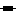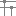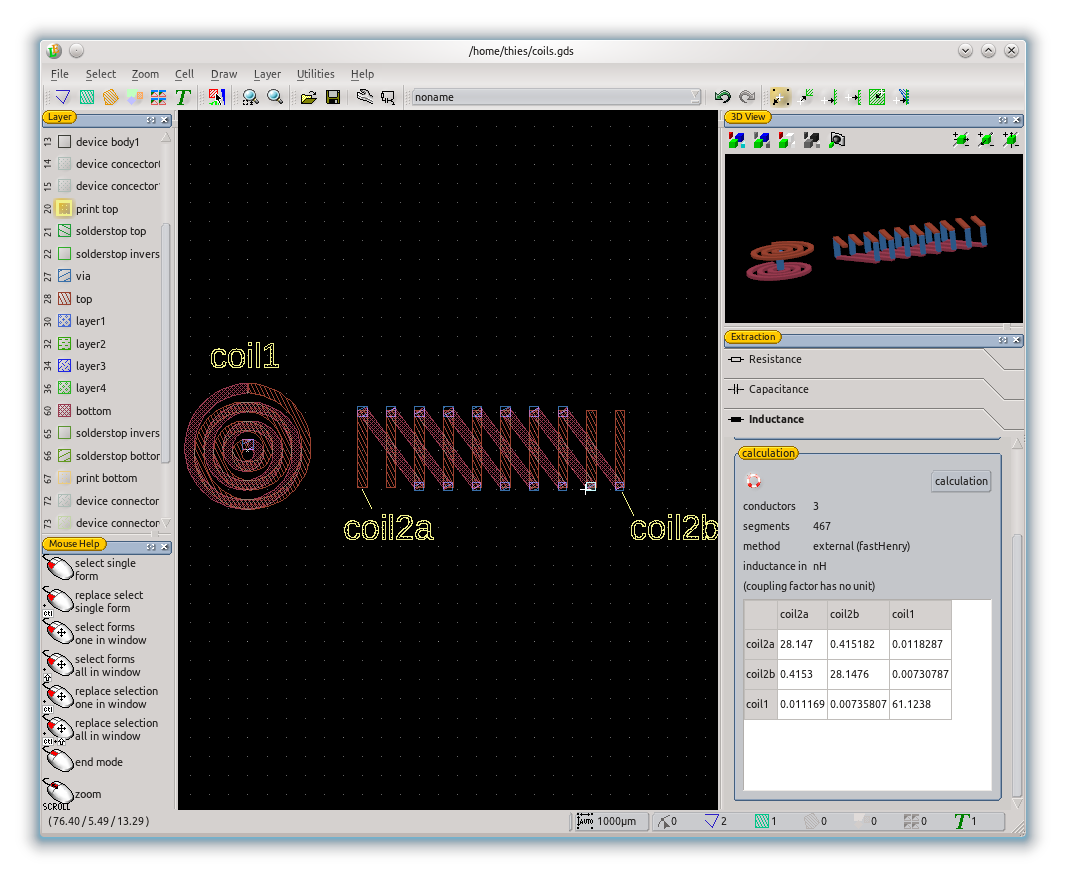# Inductance Extraction

• Icon:• Dock: Extraction

## Description

The inductance of conductors as well as the inductive coupling factor between conductors is caluculated. The calculation is three dimensional. The height value of the shapes is set in the LayerManager for each layer. The layer level, layer thickness and 2d shape define the three dimensional shape to each contuctor (see also ExtractionCapacitance and 3dView). To calculate inductance not only the conductor shape is required, but also the direction of current flow within any conductor is required. The current flow is defined by the kind of the shape. In paths a current flow is assumed along the path length and parallel to the drawing surface. In boxes and polygons the current flow is assumed perpendicular to the drawing surface. So for the example below in coil1 the two coil layers had to be drawn as paths and it contact in the middle as box or polygon. The inductance calculation is done by the external calculation tool FastHenry. FastHenry is included in the LayoutEditor package and triggered by the calculation button in the dialog.

Usage:

• select shapes for the first conductor,
• pressto add the selected shapes as a conductor,

• repeat these steps for any conductor,
• start the calculation

## Algorithm

The FastHenry algorithm is used for the calculations. It is a three dimensional calculation.

## Example## See also

ExtractionInductance (last edited 2015-01-04 11:43:13 by JürgenThies)